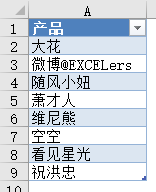# 多表查询汇总中的DAX语言应用

DAX语言是什么？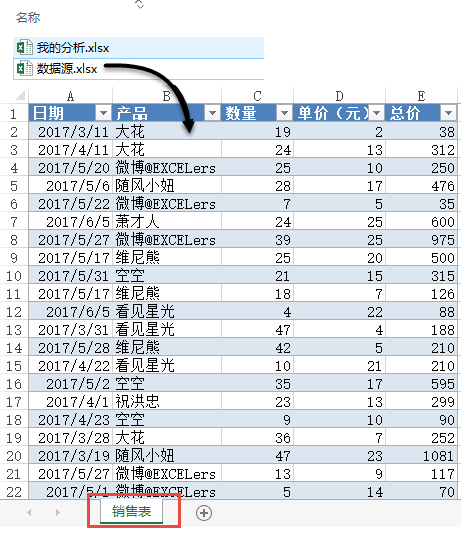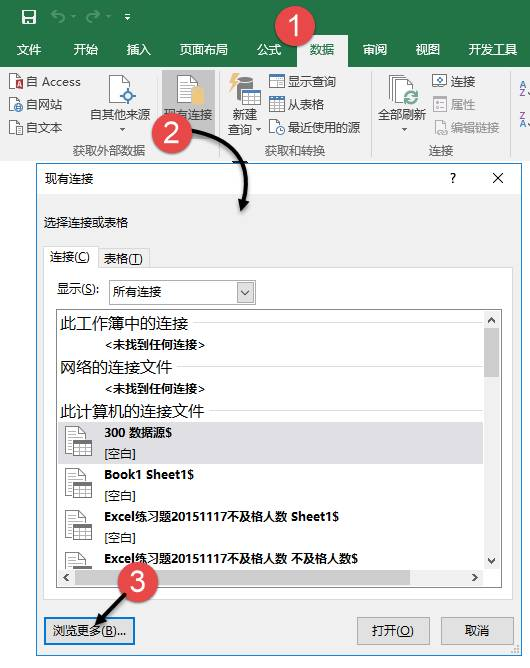【数据的放置位置】设置为现有工作表的A1单元格。单击【确定】按钮，销售表的数据将会完全读入到当前工作表中，并以“列表”的形式存在。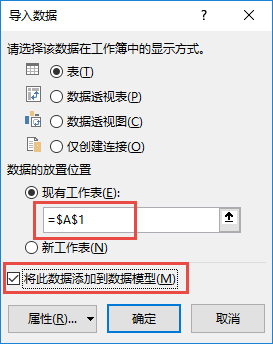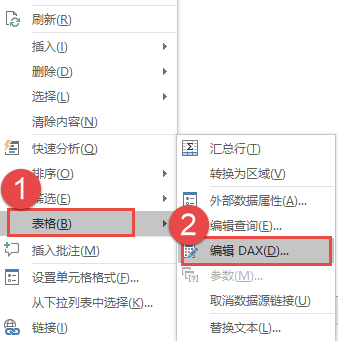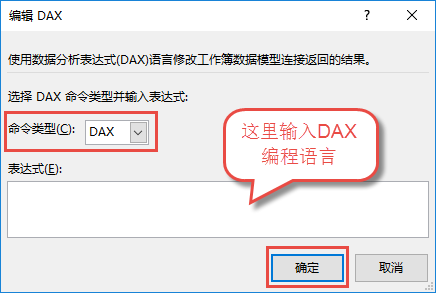（1） 字段选取。假设我们只需要获取【销售表】的“产品”和“数量”两个字段的数据。

EVALUATE

SUMMARIZE(‘销售表’,[产品],[数量])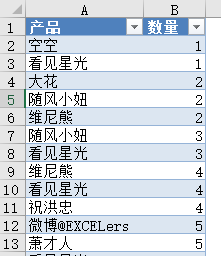（2） 条件查询。假设我们只需产品名为“大花”的销售记录。

EVALUATE

FILTER(‘销售表’,[产品]=”大花”)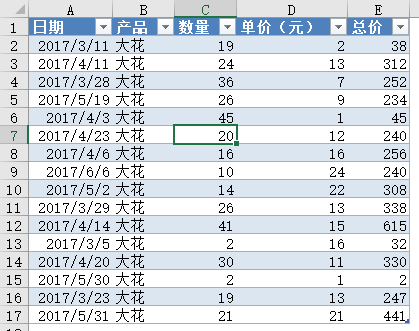（3） 汇总求和。假设我们需要获取每件商品的销售总数量。

EVALUATE

SUMMARIZE(‘销售表’,[产品],”总数量”,SUM(‘销售表'[数量]))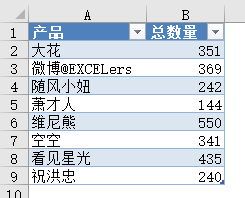（4） 条件求和。假设我们只需要获取“祝洪忠”的销售总数量。

EVALUATE

SUMMARIZE(FILTER(‘销售表’,[产品]=”祝洪忠” ),[产品],”总数量”,SUM(‘销售表'[数量]))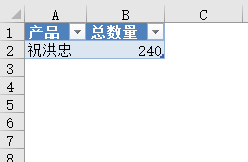（5） 汇总计数。假设我们需要获取每件商品的销售总次数。

EVALUATE

SUMMARIZE(‘销售表’,[产品],”总次数”,COUNTROWS(‘销售表’))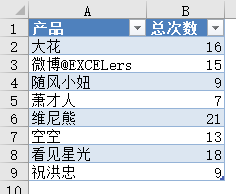（6） 条件计数。假设我们只需要获取“看见星光”的销售总次数。

EVALUATE

SUMMARIZE(FILTER(‘销售表’,[产品]=”看见星光” ),[产品],”总次数”,COUNTROWS(‘销售表’))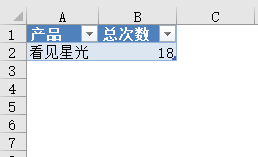（7） 名次查询。假设我们只需要获取销售总额前三名的数据，并按降序排列。

EVALUATE

SUMMARIZE(TOPN(3,’销售表’,CALCULATE(SUM(‘销售表'[总价]))),[产品],[总价]) ORDER BY [总价] DESC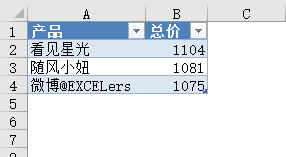（8） 去重查询。假设我们只需要提取去重复的产品名称。

EVALUATE

SUMMARIZE(‘销售表’,[产品])